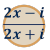Games
Problems
Go Pro!

# Complex Numbers

Reference > Mathematics > AlgebraImaginary Numbers - A brief introduction to the concepts behind imaginary numbersComplex Numbers - combining real numbers with imaginary numbers to create complex numbersHow to tell if two complex numbers are equal, by comparing real and imaginary partsHow to multiply complex numbers, how to divide and simplify complex numbersGraphing complex numbers on the complex plane, and calculating its magnitudeLike us on Facebook to get updates about new resources